Want to share your content on R-bloggers? click here if you have a blog, or here if you don't.So you know lapply, sapply, and apply…but…what about rapply, vapply, or eapply? These are generally a little less known as far as the apply family of functions in R go, so this post will explore how they work.

## rapply

Let’s start with rapply. This function has a couple of different purposes. One is to recursively apply a function to a list. We’ll get to that in a moment. The other use of rapply is to a apply a function to only those elements in a list (or columns in a data frame) that belong to a specified class. For example, let’s say we have a data frame with a mix of categorical and numeric variables, but we want to evaluate a function only on the numeric variables.

#### Use rapply to apply a function to elements of a given class

Using the traditional iris dataset, we can run the one-liner below to get the mean of each numeric column. This works almost exactly like sapply, except we add an extra parameter, class, to specify we only want to apply our function to the numeric columns in iris.

```# apply to only numeric variables
rapply(iris, mean, class = "numeric")

rapply(iris, max, class = "numeric")

rapply(iris, min, class = "numeric")

# or apply to only factor columns
rapply(iris, summary, class = "factor")

```

If you’re unsure of the class of a particular column in a data frame, df, just run the following to get the clases of each variable.

```sapply(df, class)

```

The other purpose of rapply is to apply a function recursively to a list.

```temp <- list(a = c(1, 2, 3),
b = list(a = c(4, 5, 6), b = c(7, 8, 9)),
c = list(a = c(10, 11, 12), b = c(13, 14, 15)))

rapply(temp, sum)

```Running rapply here will recursively sum the elements of each vector in each list and sub-list of temp.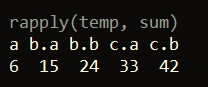rapply, similar to sapply, has an optional parameter how, which specifies how the output should be returned. So in the above example, if we wanted the output to be returned as a list instead of a vector, we could do this:

```rapply(temp, sum, how = "list")

```

Also, if our nested list contained mixed data types, we could specify applying a function to only a specific type:

```rapply(temp, sum, how = "list", class = "numeric")

```

## vapply

vapply works similarly to sapply, except that it requires an extra parameter specifying the type of the expected return value. This extra parameter is useful in coding because it can help ensure silent errors don’t cause issues for you.

For example, suppose we have the following list of mixed data types:

```sample_list <- list(10, 20, 30, "some_string")

```

Now if we run the below code with sapply, we get a vector of characters, which is not exactly what we want. For instance, if we didn’t know our list had mixed data types, and we ran our function to the get the max of each element in the list, then R doesn’t return an error here. Instead, it silently converts the output to a character vector.

```sapply(sample_list, max)

```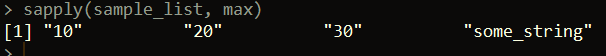However, we can catch this error using vapply.

```vapply(sample_list, max, numeric(1))

```

This third parameter, numeric(1) specifies that we want the output returned by vapply to be numeric. This means if an issue occurs in returning a numeric result, vapply will result in an error, rather than trying to coerce the result to a different data type. This could allow you to investigate why a character is in the list, for example.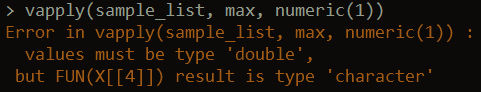vapply’s ability to catch errors based off data types makes it useful for running R code in production or as a scheduled task as an extra measure to guard against type issues.

## eapply

eapply applies a function to every named element in an environment. This requires a little knowledge about how environments work in R. It works pretty similarly to lapply in that it also returns a list as output. The input to eapply, however, must be an environment, whereas lapply can take a variety of objects as inputs.

Here’s an example:

```# create a new environment
e <- new.env()

e\$a <- 10
e\$b <- 20
e\$c <- 30

# multiply every element in the environment by 2
new_e <- eapply(e, function(x) x * 2)

```

In the above example, we create a list from the initiated environment, e, and then double the value of each element.

Here’s another example, using the environment within a function:

```sample_func <- function()
{

df1 <- data.frame(a = c(1, 2, 3, 4), b = c(5, 6, 7, 8))
df2 <- data.frame(a = c(1, 2, 3, 4, 5), b = c(5, 6, 7, 8, 9))
df3 <- data.frame(a = c(1, 2, 3, 4, 5, 6), b = c(5, 6, 7, 8, 9, 10))

eapply(environment(), nrow)

}

sample_func()

```

Above, calling sample_func will return a list of the number of rows for each respective data frame defined within the function’s environment: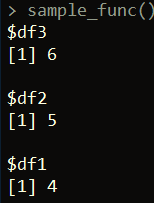eapply can also be called with the parameter USE.NAMES = FALSE, which will return an unnamed list.

```eapply(environment(), nrow, USE.NAMES = FALSE)

```

One other difference versus lapply is that eapply can take an optional Boolean parameter called all.names that specifies if the function input should be applied to just the visible elements of the environment, or to all objects. Here’s an example to illustrate:

```e <- new.env()
e\$.test <- 100
e\$other_test <- 64

```

Here, we defined an environment with one object called .test and one called other_test. Next, if we run this:

```eapply(e, sqrt)

```

we’ll get back a list with one element – 8. As in, eapply is only applying the sqrt function to the object named other_test in e because it is not a hidden object. Names that begin with a dot (hidden objects) are excluded from the function’s application. To apply the function to every object in the environment, we need to set the parameter all.names = TRUE.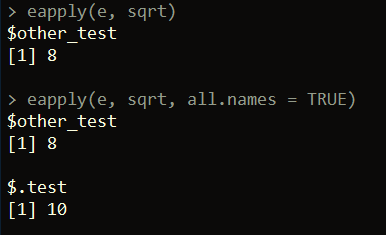Please check out other R articles of mine here: http://theautomatic.net/category/r/.

The post Those “other” apply functions… appeared first on Open Source Automation.